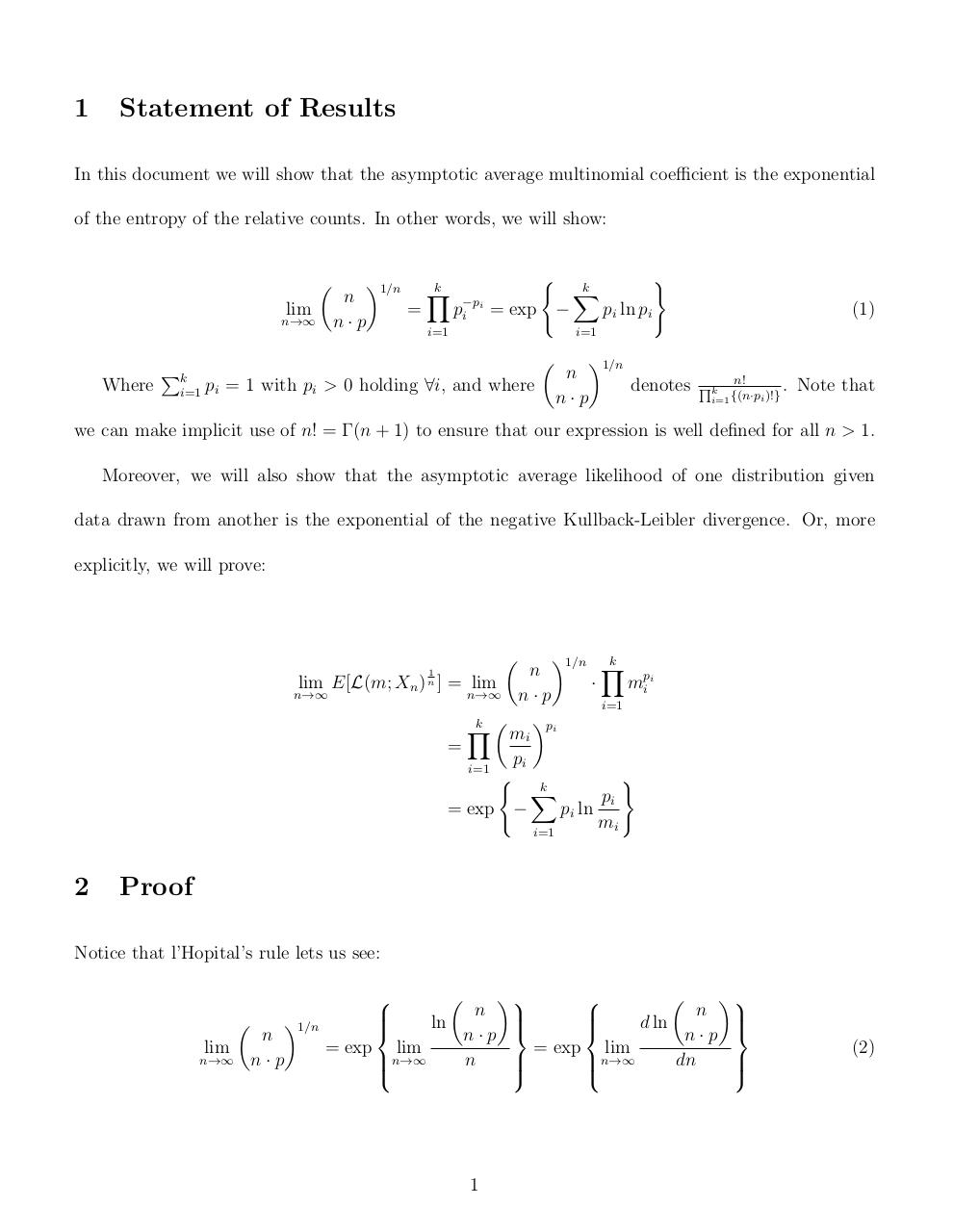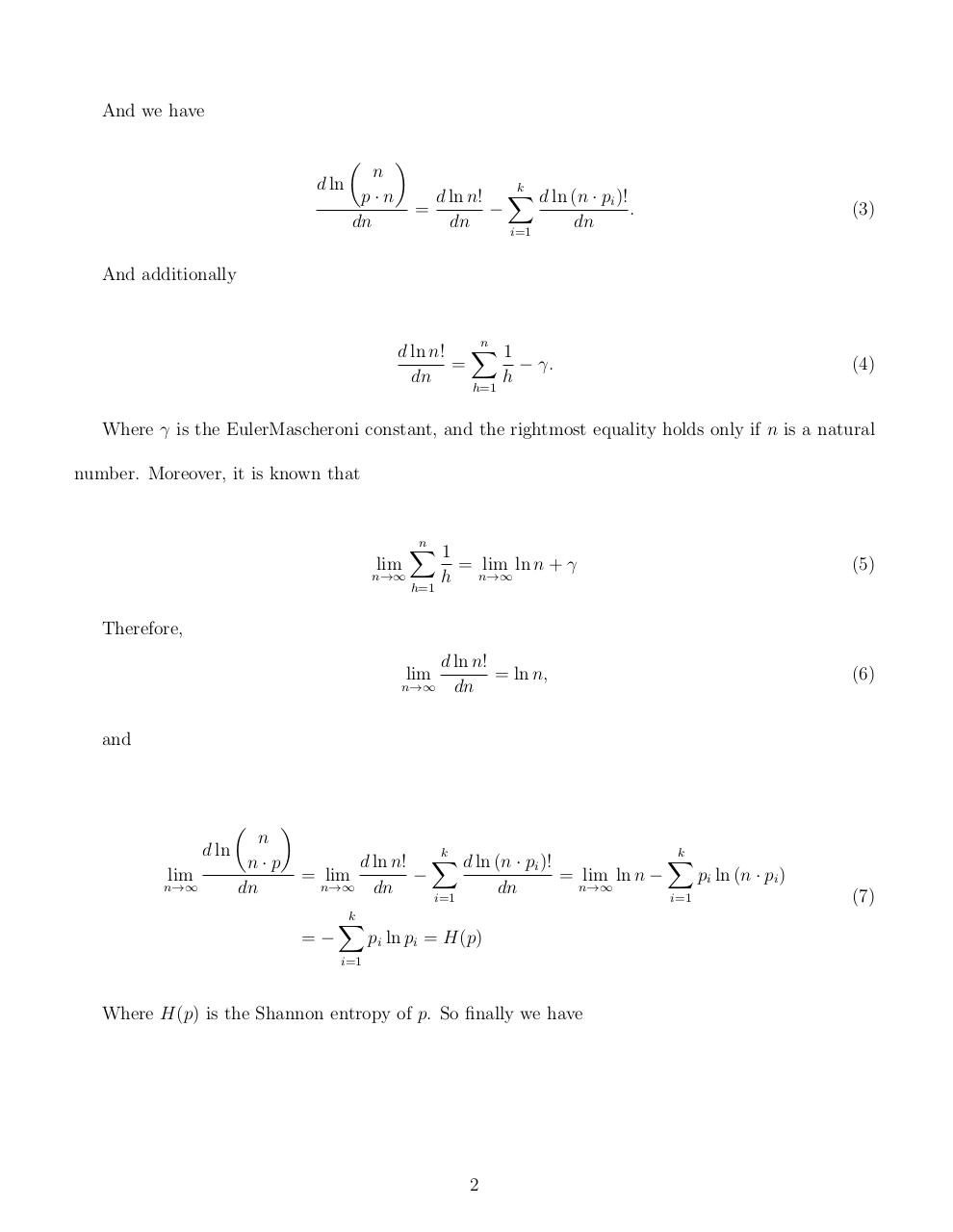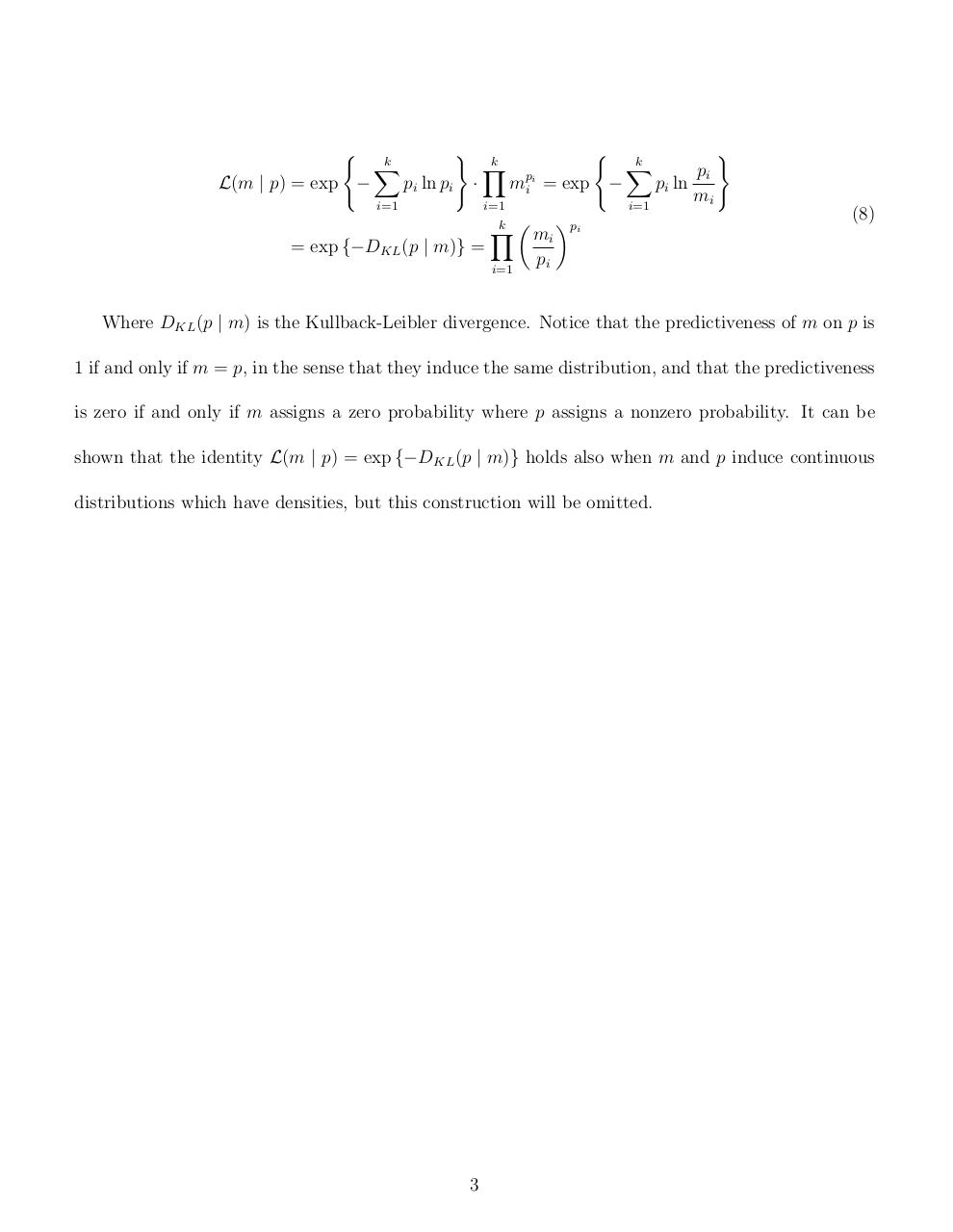# pftheorm1 .pdf

### File information

Original filename: pftheorm1.pdf

This PDF 1.5 document has been generated by TeX / MiKTeX pdfTeX-1.40.12, and has been sent on pdf-archive.com on 30/05/2013 at 22:51, from IP address 142.157.x.x. The current document download page has been viewed 728 times.
File size: 116 KB (3 pages).
Privacy: public file

## Download original PDF file

pftheorm1.pdf (PDF, 116 KB)

### Document preview

1

Statement of Results

In this document we will show that the asymptotic average multinomial coefficient is the exponential
of the entropy of the relative counts. In other words, we will show:

lim

n→∞

Where

Pk

n
n·p

1/n
=

k
Y

(
i
p−p
= exp −
i

i=1

)
pi ln pi

(1)

i=1

i=1

k
X

pi = 1 with pi &gt; 0 holding ∀i, and where

n
n·p

1/n
denotes

n!
Qk

i=1 {(n·pi )!}

. Note that

we can make implicit use of n! = Γ(n + 1) to ensure that our expression is well defined for all n &gt; 1.
Moreover, we will also show that the asymptotic average likelihood of one distribution given
data drawn from another is the exponential of the negative Kullback-Leibler divergence. Or, more
explicitly, we will prove:

1/n Y
k
n
lim E[L(m; Xn ) ] = lim
·
mpi i
n→∞
n→∞ n · p
i=1
pi
k
Y
mi
=
pi
i=1
( k
)
X
pi
= exp −
pi ln
mi
i=1

1
n

2

Proof

Notice that l’Hopital’s rule lets us see:

lim

n→∞

n
n·p

1/n

n
ln
n·p
= exp lim
n→∞

n

1

n
d ln
n·p
= exp lim
n→∞

dn

(2)

And we have

n
d ln
p·n
dn

k

d ln n! X d ln (n · pi )!
=

.
dn
dn
i=1

(3)

And additionally

n

d ln n! X 1
=
− γ.
dn
h
h=1

(4)

Where γ is the EulerMascheroni constant, and the rightmost equality holds only if n is a natural
number. Moreover, it is known that

n
X
1
lim
= lim ln n + γ
n→∞
n→∞
h
h=1

(5)

d ln n!
= ln n,
n→∞ dn

(6)

Therefore,
lim

and

n
d ln
n·p
lim
n→∞
dn

k

k

X
d ln n! X d ln (n · pi )!
= lim

= lim ln n −
pi ln (n · pi )
n→∞ dn
n→∞
dn
i=1
i=1
=−

k
X

pi ln pi = H(p)

i=1

Where H(p) is the Shannon entropy of p. So finally we have

2

(7)

(
L(m | p) = exp −

k
X

)
pi ln pi

·

i=1

k
Y

(
mpi i

i=1
k
Y

= exp {−DKL (p | m)} =

i=1

= exp −

k
X
i=1

mi
pi

pi

pi
pi ln
mi

)
(8)

Where DKL (p | m) is the Kullback-Leibler divergence. Notice that the predictiveness of m on p is
1 if and only if m = p, in the sense that they induce the same distribution, and that the predictiveness
is zero if and only if m assigns a zero probability where p assigns a nonzero probability. It can be
shown that the identity L(m | p) = exp {−DKL (p | m)} holds also when m and p induce continuous
distributions which have densities, but this construction will be omitted.

3### Link to this page

#### Permanent link

Use the permanent link to the download page to share your document on Facebook, Twitter, LinkedIn, or directly with a contact by e-Mail, Messenger, Whatsapp, Line..

#### Short link

Use the short link to share your document on Twitter or by text message (SMS)

#### HTML Code

Copy the following HTML code to share your document on a Website or Blog

#### QR Code### Related keywords